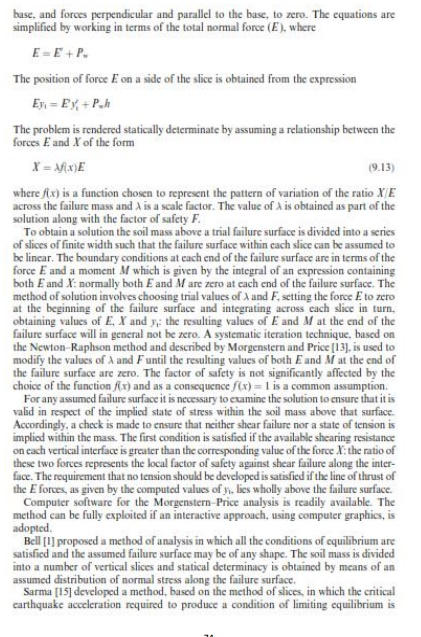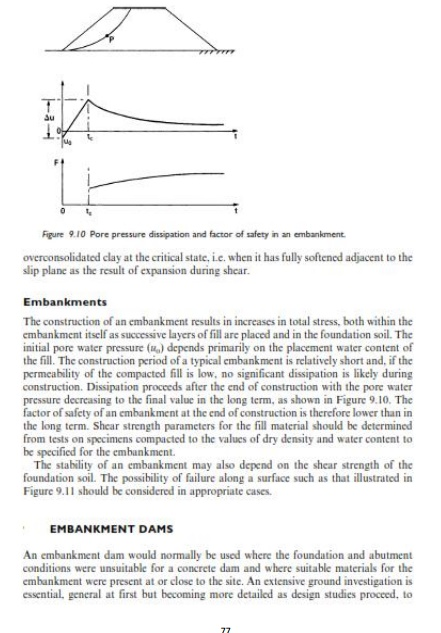Home | | Soil Mechanics | Soil Mechanics: Slope Stability

# Soil Mechanics: Slope StabilityThe Method OF Slices: In this method the potential failure surface, in section, is again assumed to be a circular arc with centre O and radius r.

The Method OF Slices

In this method the potential failure surface, in section, is again assumed to be a circular arc with centre O and radius r. The soil mass (ABCD) above a trial f ailure surface (AC)  is divided  by  ve rtical  planes  into         a  series of  slices of  width  b, as  shown  in Figure      9.5. The base  of each slice is assumed to be a straight line. For any slice the inclination of the base to the horizontal is and the height, measur ed on the centre- line, is h. The analysis is based on the use of a lumped factor of safety (F ), defined as the ratio of the available shear strength ( ) to the shear strength (Tm) which must be mobilized to maintain a condition of limiting equilibrium, i.e.

F=Pi/TmThe factor of safety is taken to be the same for each slice, implyi ng that there must be mutual support betweenn slices, i.e. forces must act between the slices.

The forces (per unit dim ension normal  to the section) acting on a sli ce are:Study Material, Lecturing Notes, Assignment, Reference, Wiki description explanation, brief detail
Civil : Soil Mechanics -Slope Stability : Soil Mechanics: Slope Stability |